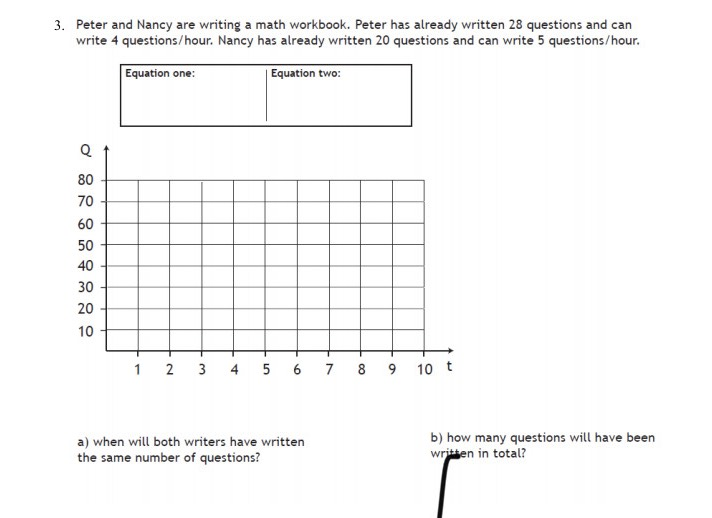### Still have math questions?

Algebra
QuestionPeter and Nancy are writing a math workbook. Peter has already written $$28$$ questions and can write $$4$$ questions/hour. Nancy has already written $$20$$ questions and can write $$5$$ questions/hour.

a) when will both writers have written the same number of questions?

b) how many questions will have been written in total?